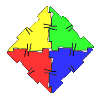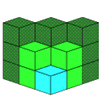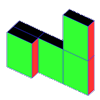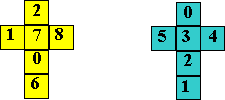#### You may also like### Polydron

This activity investigates how you might make squares and pentominoes from Polydron.If you had 36 cubes, what different cuboids could you make?### Cereal Packets

How can you put five cereal packets together to make different shapes if you must put them face-to-face?

# Calendar Cubes

##### Age 7 to 11Challenge Level

Jessica from Tattingstone School has explained how she solved this problem.

I found out that you could fit 6 numbers on each cube so that would mean I had to find the twelve most important. Then I worked there were only 9 different numbers in the month. Then I worked out I would need to use 1, 2, 3 more than once so 9 + 3 + 12 and that is the correct amount of numbers needed.

Then I found out that I needed to have a 1, 2, 0 on each one. I didn't need a 3 because there's no such number as 33 in the year. I needed a 0 on each one for 10 and 01.George from Rosebank Primary School, Leeds has sent in an excellent solution. He has thought about the problem in a similar way to Jessica and worked out which numbers are essential and which cube they need to be on. He says:

I noticed that I could not have the numbers from 4 to 9 on one of the cubes and on the other cube from 0 to 3, 4 and 5 because there wouldn't be the 10th, the 11th, the 12th, the 13th, the 01st, the 02nd, the 03rd, the 20th, the 21st, the 22nd, the 23rd. That will happen because there isn't 2 or 1 on both cubes.

So what I did was I made the cubes have 0, 1, 2, and 1 of them have 3. Then I made 1 of the cubes have 4 and 5 which was the one which had the 3. After that I was left with the numbers 6, 7, 8, 9 but the 9 could have been made to a 6 so I didn't have 9 on my 2nd cube but I had 6, 7, 8.

Hayley from Bradpole Symonsbury School also had of the idea of turning the 9 and 6 upside down.